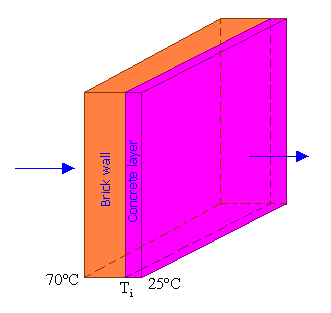### Heat Loss by Conduction

A plane brick wall, 25 cm thick, is faced with 5 cm thick concrete layer. If the temperature of the exposed brick face is 70oC and that of the concrete is 25oC, find out the heat lost per hour through a wall of 15 m x10 m. Also, determine the interface temperature. Thermal conductivity of the brick and concrete are 0.7 W/m.K and 0.95 W/m.K respectively.

Calculations:Heat loss Q = (Ta - Tb) / R

Where Ta = 70oC; Tb = 25oC; and

R = Rbrick + Rconcrete

Rbrick = Lbrick/(Akbrick)

Rconcrete = Lconcrete/(Akconctrete)

A = 15 x 10 = 150 m2

Rbrick = 0.25/(150 x 0.7) = 2.381 x 10-3 oC/W

Rconcrete = 0.05/(150 x 0.95) = 3.509 x 10-4 oC/W

R = 2.381 x 10-3 + 3.509 x 10-4 = 2.732 x 10-3 oC/W

Q = (70 - 25) / (2.732 x 10-3) = 16471.4 W = 16471.4 J/sec = 59.3 MJ/hr

Heat loss per hour = 59.3 MJ

At steady state, this is the amount of heat transferred through the brick wall or concrete per hour.

i.e.,

Q = A kbrick(Ta - Ti)/Lbrick = 16471.4 W

150 x 0.7 x (70 - Ti) / 0.25 = 16471.4

70 - Ti = 39.2

Ti = 70 - 39.2 = 30.8oC

Interface temperature Ti = 30.8oC